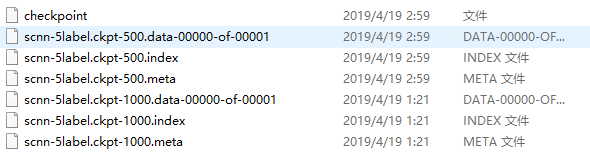• ## pytorch模型保存

千次阅读 2018-03-04 10:15:30
pytorch模型保存方式： pytorch官网手册 torchvision.models中的下载下来的pretrained模型，比如：alexnet-owt-4df8aa71.pth，是将模型的参数，按照有序字典的方式保存。类型为：collections.OrderedDict 方式...
#pytorch模型保存方式： pytorch官网手册
torchvision.models中的下载下来的pretrained模型，比如：alexnet-owt-4df8aa71.pth，是将模型的参数，按照有序字典的方式保存。类型为：collections.OrderedDict
###方式一：仅保存和加载模型参数（推荐的方式）
1 . 保存模型参数：
import torch
torch.save(model.state_dict(), 'save_path_name.pth') # 后缀不重要(torchvision.models下载下来的模型参数后缀为.pth)

2 . 加载模型参数：
import torch
import torch.nn as nn

例子：
import torch
import torch.nn as nn
import torchvision
import AlexNet_Train_Val

model = AlexNet_Train_Val.ModifiedAlexNet(2)

###方式二：保存和加载整个模型(模型结构和模型参数) 1 . 保存模型：
import torch
torch.save(model, 'save_path_name.pth')

2 . 加载模型：
import torch
import torch.nn as nn

额外的参考：https://zhuanlan.zhihu.com/p/82038049
展开全文pytorch
• ## Pytorch模型保存

千次阅读 2019-03-14 14:30:07
Pytorch 模型保存和加载保存和加载整个模型保存加载模型参数 保存和加载整个模型 torch.save(modelname, 'model.pkl') model = torch.load('model.pkl') 保存加载模型参数 torch.save(modelname.state_dict()...


Pytorch 模型保存和加载
保存和加载整个模型保存加载模型参数

保存和加载整个模型
torch.save(modelname, 'model.pkl')

该方式无需自定义网络模型，保存时已把网络结构保存，不方便调整网络结构 。
保存加载模型参数
torch.save(modelname.state_dict(), 'params.pkl')

该方式需要自己定义网络，并且其中的参数名称与结构要与保存的模型中的一致（可以定义部分网络，比如只使用VGG的前几层），相对灵活，便于对网络进行修改。
 https://blog.csdn.net/u012436149/article/details/68948816  https://blog.csdn.net/u011276025/article/details/78507950
展开全文Pytorch 深度学习
• TF模型保存 测试篇 可参考TensorFlow之CNN图像分类及模型保存与调用 和『TensorFlow』模型保存和载入方法汇总和模型的保存与恢复(Saver) 模型保存——saver model_path='E:/model/kdd/model.ckpt' ##绝对路径 ...
TF模型保存 测试篇
可参考TensorFlow之CNN图像分类及模型保存与调用 和『TensorFlow』模型保存和载入方法汇总和模型的保存与恢复(Saver)
模型保存——saver
  model_path='E:/model/kdd/model.ckpt' ##绝对路径
model_path='scnn_model/model.ckpt'##相对路径
tf.train.Saver.save(sess,model_path)
saver=tf.train.Saver()

调用模型进行预测
可以先看模型保存了哪些文件.meta文件保存了当前图结构
.data文件保存了当前参数名和值
.index文件保存了辅助索引信息
.data文件可以查询到参数名和参数值，使用下面的命令可以查询保存在文件中的全部变量{名：值}对，

tf.train.get_checkpoint_state( )函数可以通过检查点文件锁定最新的模型 tf.train.import_meta_graph( )函数给出model.ckpt-n.meta的路径后会加载图结构，并返回saver对象 saver.restore(sess, model.model_checkpoint_path)用于模型的恢复
ckpt = tf.train.get_checkpoint_state('ckpt-5/') # 通过检查点文件锁定最新的模型
saver = tf.train.import_meta_graph(ckpt.model_checkpoint_path + '.meta')  # 载入图结构，保存在.meta文件中
saver.restore(sess, ckpt.model_checkpoint_path)

tf.train.Saver函数会返回加载默认图的saver对象，saver对象初始化时可以指定变量映射方式，根据名字映射变量 下面是最近一个完整的加载过程
# 加载模型
saver = tf.train.Saver()
with tf.Session() as sess:
ckpt = tf.train.get_checkpoint_state('ckpt-5/')  # 通过检查点文件锁定最新的模型
saver = tf.train.import_meta_graph(ckpt.model_checkpoint_path + '.meta')  # 载入图结构，保存在.meta文件中
if ckpt and ckpt.model_checkpoint_path:
global_step = ckpt.model_checkpoint_path.split('/')[-1].split('-')[-1]
saver.restore(sess, ckpt.model_checkpoint_path)
else:
print('No Checkpoint')
#ii=0
graph = tf.get_default_graph()
xs = graph.get_tensor_by_name("inputs/pic_data:0")
keep_prob = graph.get_tensor_by_name("inputs/keep_prob:0")
logits = graph.get_tensor_by_name("prediction_eval:0")
prediction = sess.run(logits, feed_dict={xs: feature,keep_prob: 1.0})  ##?
print('Prediction Matrix of Test Data Set:')
print(prediction)
max_index = np.argmax(prediction, 1)
print('Prediction Vector of Test Data Set:')
print(max_index)
m0 = max_index
print('Size of Test Data Set:   ',m0.shape)


上述过程，调用了模型中graph中的tensor，注意大小要保持一致
xs = graph.get_tensor_by_name("inputs/pic_data:0")#我的命名空间input中有一个名为pic_data的tensor

如果不知道原图中有哪些tensor，可以加载模型后按照下面语句去查看
#获得几乎所有的operations相关的tensor
ops = [o for o in sess.graph.get_operations()]
for o in ops:
print(o.name)

最后奉上
模型的干货加载
# 连同图结构一同加载
ckpt = tf.train.get_checkpoint_state('./model/')
saver = tf.train.import_meta_graph(ckpt.model_checkpoint_path +'.meta')
with tf.Session() as sess:
saver.restore(sess,ckpt.model_checkpoint_path)

# 只加载数据，不加载图结构，可以在新图中改变batch_size等的值
# 不过需要注意，Saver对象实例化之前需要定义好新的图结构，否则会报错
saver = tf.train.Saver()
with tf.Session() as sess:
ckpt = tf.train.get_checkpoint_state('./model/')
saver.restore(sess,ckpt.model_checkpoint_path)


展开全文TensorFlow
• ## TF2.0模型保存

千次阅读 2020-02-23 20:20:43
TF2.0模型保存概述模型保存 概述 这是TF2.0入门笔记【TF2.0模型创建、TF2.0模型训练、TF2.0模型保存】中最后一篇【TF2.0模型保存】、前两篇分别介绍了模型的创建与训练，本篇将介绍模型的保存以及加载。 模型保存 ...


TF2.0模型保存
概述模型保存及加载保存为.h5格式1、保存权重2、加载权重3、保存整个模型4、加载模型
保存为tf格式保存为.pb格式（Saved Model）1、保存成Saved Model2、加载Saved Model

完结撒花

概述
这是TF2.0入门笔记【TF2.0模型创建、TF2.0模型训练、TF2.0模型保存】中最后一篇【TF2.0模型保存】，前两篇分别介绍了模型的创建与训练，本篇将介绍模型的保存以及加载。
模型保存及加载
事实上，tensorflow1.x和tensorflow2.0的模型保存略有差别，tensorflow1.x还有一个freeze_graph的步骤…。但是这里就不做讨论了，本篇仅以TF2.0作为介绍。
import tensorflow as tf
import os
from tensorflow.keras.layers import Dense, Flatten, Conv2D
from tensorflow.keras import Sequential

model = Sequential()

mnist = tf.keras.datasets.mnist

(x_train, y_train), (x_test, y_test) = mnist.load_data()
x_train, x_test = x_train / 255.0, x_test / 255.0

x_train = x_train[..., tf.newaxis]
x_test  = x_test[..., tf.newaxis]

loss='sparse_categorical_crossentropy',
metrics=['accuracy'])

保存为.h5格式
1、保存权重
保存方式可以通过callback保存，也可以在最后通过调用save_weights方法保存 1.1、通过callback保存
model_path = "./model"
model_name = "weight_callback.h5"
if not os.path.exists(model_path):
os.mkdir(model_path)

model_file = os.path.join(model_path, model_name)
callback = tf.keras.callbacks.ModelCheckpoint(filepath=model_file,
save_weights_only=True,
verbose=1)

model.fit(x_train, y_train, epochs=1, callbacks=[callback])

1.2、在最后调用save_weights方法保存
model.save_weights('./model/weight_save.h5')

运行上面的两个代码，我们会得到以下两个权重文件：2、加载权重
接下来我们加载一下看看它们测试结果是不是一样的。 注意：由于是我们保存的是模型的参数，所以我们加载的时候需要需要重新定义模型结构
model = Sequential()

loss='sparse_categorical_crossentropy',
metrics=['accuracy'])

2.1、加载使用callback方法保存的权重
model.load_weights('./model/weight_callback.h5')
model.evaluate(x_test,  y_test, verbose=2)

运行输出：
10000/10000 - 2s - loss: 0.0644 - accuracy: 0.9781
[0.06437707488704472, 0.9781]

2.2、加载使用save_weights方法保存的权重
model.load_weights('./model/weight_save.h5')
model.evaluate(x_test,  y_test, verbose=2)

运行输出：
10000/10000 - 2s - loss: 0.0644 - accuracy: 0.9781
[0.06437707488704472, 0.9781]

分析：我们可以看到，结果是一样的
3、保存整个模型
保存整个模型跟保存权重一样，也是两种方法。 1、通过callback保存 这里把save_weights_only改成False就可以了
model_path = "./model"
model_name = "model_callback.h5"
if not os.path.exists(model_path):
os.mkdir(model_path)

model_file = os.path.join(model_path, model_name)
callback = tf.keras.callbacks.ModelCheckpoint(filepath=model_file,
save_weights_only=False,
verbose=1)

model.fit(x_train, y_train, epochs=1, callbacks=[callback])

2、在最后调用save方法保存
model.save('./model/model_save.h5')

运行上面的两个代码，我们会得到以下两个模型文件：4、加载模型
model = load_model('./model/model_callback.h5')
model.evaluate(x_test,  y_test, verbose=2)

运行输出：
10000/10000 - 2s - loss: 0.0602 - accuracy: 0.9814
[0.0602496856124606, 0.9814]

4.2、加载使用save方法保存的模型
model = load_model('./model/model_save.h5')
model.evaluate(x_test,  y_test, verbose=2)

运行输出：
10000/10000 - 2s - loss: 0.0602 - accuracy: 0.9814
[0.0602496856124606, 0.9814]

保存为tf格式
事实上，TF2.0已经不推荐或者说不支持将参数保存为.ckpt格式了，这里演示的也不能说是.ckpt格式，官方文档称之为’tf’格式，如果你不指定是’h5’格式，那它就是’tf’格式。（个人不推荐使用这种方法，事实上这里我也不太懂）
model_path = "./model"
model_name = "weight_callback.ckpt"
if not os.path.exists(model_path):
os.mkdir(model_path)

model_file = os.path.join(model_path, model_name)
callback = tf.keras.callbacks.ModelCheckpoint(filepath=model_file,
save_weights_only=True,
verbose=1)

model.fit(x_train, y_train, epochs=1, callbacks=[callback])

运行得到以下文件： 这里只要你文件名的后缀不是.h5（如果后缀不是.h5，那么就会保存成’tf’格式，这时后缀已经变得不重要了，你甚至可以不要后缀），那么保存之后你就会得到以下三个文件。model.load_weights('./model/weight_callback.ckpt')
model.evaluate(x_test,  y_test, verbose=2)

运行输出：
10000/10000 - 2s - loss: 0.0637 - accuracy: 0.9789
[0.06373853980554267, 0.9789]

保存为.pb格式（Saved Model）
1、保存成Saved Model
这里也仍然有两种方式将模型保存为Saved Model格式。 1.1、通过callback保存 想要通过callback保存成Saved Model格式，必须要传递的是一个文件夹，而不是文件名，而且save_weights_only=False这个参数一定要设成False，不然就会保存为之前说的’tf’格式，注意：这两个条件缺一不可。
model_path = "./model"

if not os.path.exists(model_path):
os.mkdir(model_path)

callback = tf.keras.callbacks.ModelCheckpoint(filepath=model_path,
save_weights_only=False,
verbose=1)

1.2、在最后用tf.saved_model.save方法保存
tf.saved_model.save(model, './model')

运行上面的两个代码，我们会得到以下文件（两种方法运行结果一样）：2、加载Saved Model
model = load_model('./model/')
model.evaluate(x_test,  y_test, verbose=2)

运行输出：
10000/10000 - 2s - loss: 0.0663 - accuracy: 0.9790
[0.06630929337199777, 0.979]

完结撒花
TF2.0入门笔记【TF2.0模型创建、TF2.0模型训练、TF2.0模型保存】三部曲
展开全文tensorflow 深度学习 人工智能 机器学习 python
• ## TensorFlow模型保存和提取方法

万次阅读 多人点赞 2017-06-01 11:25:25
一、TensorFlow模型保存和提取方法 1. TensorFlow通过tf.train.Saver类实现神经网络模型的保存和提取。tf.train.Saver对象saver的save方法将TensorFlow模型保存到指定路径中，saver.save(sess,"Model/model.ckpt")...TensorFlow 模型提取
• 主要介绍了浅谈tensorflow模型保存为pb的各种姿势，具有很好的参考价值，希望对大家有所帮助。一起跟随小编过来看看吧
• 前言：tensorflow2.0中有很多种模型保存方法，为了做一个统一的比较，本文做一个归纳总结，加之自己在这上面踩过很多的坑，现在感觉做一个总结实在是有必要。因为tensorflow创建模型的方式很多，包括Sequence、Model...tensorflow keras checkpoint
• 模型保存读取参考 TensorFlow 模型保存/载入的两种方法 TensorFlow保存或加载训练的模型 模型参数保存 保存时会把你里边定义的所有变量保存，W和b都会保存 import tensorflow as tf import numpy as np W = ...TensorFlow
• keras模型保存和加载与tensorflow不同，keras中的模型保存和加载通常是保存成hdf5格式。 keras的模型保存分为多种情况。 一、不保存模型只显示大概结构 model.summary() 这个函数会打印模型结构，但是仅仅是...
• python训练好的模型保存与加载 当我们训练好一个模型model后，如果在其他程序中或者下次想继续使用这个model，我们就需要把这个model保存下来，下次使用时直接导入就好了，不需要重新训练。 方式一： 采用joblib模块...
• 1 模型保存 TensorFlow提供了一个非常方便的api，tf.train.Saver()来保存和还原一个机器学习模型。程序会生成并保存四个文件: checkpoint 文本文件，记录了模型文件的路径信息列表 mnist-10000.data-00000-of-...
• ## 模型保存与使用

千次阅读 2018-03-20 21:14:41
tf里面提供模型保存的是tf.train.Saver()模块。模型保存，先要创建一个Saver对象：如saver=tf.train.Saver() max_to_keep 参数，这个是用来设置保存模型的个数，默认为5，即 max_to_keep=5，保存最近的5个模型。...
• tensorflow+cnn神经网络学习模型保存及调用，通过五种类型花卉(附在附件中）真实jpg图片，实现数据的分类学习，模型的保存，及调用方法。
• 一、TensorFlow模型保存和提取方法 1. TensorFlow通过tf.train.Saver类实现神经网络模型的保存和提取。tf.train.Saver对象saver的save方法将TensorFlow模型保存到指定路径中，saver.save(sess,"Model/model....tensorflow model
• 1 原生模式 # 模型训练 gbm = lgb.train(params, lgb...# 模型保存 gbm.save_model('model.txt') # 模型加载 gbm = lgb.Booster(model_file='model.txt') # 模型预测 y_pred = gbm.predict(X_test, num_iteration=.
• ## PyTorch模型保存与加载

万次阅读 多人点赞 2019-05-28 14:58:10Pytorch
• import shelve class A: def __init__(self,name,a): self.name=name self.a=a def rewrite(self,args): self.a=args obj1=A("qw","1") obj2=A("qa","2") obj3=A("qs","3") #写入文件 db=shelve.open("userinfo...kotlin
• python中归一化、标准化模型保存与加载，python中归一化、标准化模型保存与加载归一化
• pytorch的pt模型保存 仅保存和加载模型参数 # 模型保存 checkpoint={'epoch':epoch, 'best_loss':best_loss, 'model':model.state_dict() 'optimizer':optimizer.state_dict() } torch.save(checkpoint, ...pytorch
• 如果你从我的这篇博客http://blog.csdn.net/j56754gefge/article/details/40708679里下载了DPM目标检测代码，它所使用的模型文件是保存在txt里的，而MATLAB训练出来的模型是mat文件，该函数提供将MATLAB模型保存为...
• Keras框架训练模型保存及再载入 实验数据MNIST 初次训练模型并保存 import numpy as np from keras.datasets import mnist from keras.utils import np_utils from keras.models import Sequential from keras....
• 话不多说，干就完了。 变量重命名的用处？ 简单定义：简单来说就是将模型A中的参数parameter_A赋给模型B中的parameter_B 使用场景：当需要使用已经训练好的模型参数，尤其是使用...1）、模型保存 import os im...tensorflow 变量重命名
• 一、 sklearn中提供了高效的模型持久化模块joblib，将模型保存至硬盘。 from sklearn.externals import joblib #lr是一个LogisticRegression模型 joblib.dump(lr, 'lr.model') lr = joblib.load('lr.model') 二、...
• TensorFlow模型保存和加载方法 模型保存 import tensorflow as tf w1 = tf.Variable(tf.constant(2.0, shape=), name="w1-name") w2 = tf.Variable(tf.constant(3.0, shape=), name="w2-name") a = tf....TensorFlow
• ## tensorflow模型保存为pb的各种姿势

万次阅读 多人点赞 2019-03-15 19:32:41
tensorflow模型保存的各种姿势 一，直接保存pb 1, 首先我们当然可以直接在tensorflow训练中直接保存为pb为格式，保存pb的好处就是使用场景是实现创建模型与使用模型的解耦，使得创建模型与使用模型的解耦，使得前...
• TensorFlow2教程-keras模型保存和序列化 1.保持序列模型和函数模型 # 构建一个简单的模型并训练 from __future__ import absolute_import, division, print_function import tensorflow as tf tf.keras.backend...教程 入门 keras 序列化...